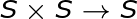# Rings: Binary Structures & Ring Homomorphism

Lesson Transcript
Instructor: Russell Frith
In this lesson, basic definitions are presented for algebraic rings and ring homomorphisms. These form the foundations for a broader discourse in abstract algebra. Rings are used in more complex algebraic structures and have many applications in computer science, physics, and engineering.

## Rings

Let's say you are in Honolulu and you want to call your friend in Tokyo. Your local time is 11:45 AM and you know Tokyo is 19 hours ahead of you. If you call your friend, will she be awake or not?(11:45 AM plus 19 hours makes the time in Tokyo to be 6:45 AM. When you add 19 hours, you're performing an arithmetic calculation known as clock arithmetic.

This type of addition is explained algebraically using the notion of rings. Let S be a set which is a container holding an unspecified number of named objects designated as a, b, c, and so on. The elements of the set may be subjected to set operations such as addition and multiplication. When the elements of a set are transformed by some set operation, the result of the transformation is also an existing element within the set. If the result of the set operation generates a result which is not in the given set, then the set is not a ring.

An error occurred trying to load this video.

Try refreshing the page, or contact customer support.

Coming up next: How to Write Sets Using Set Builder Notation

### You're on a roll. Keep up the good work!

Replay
Your next lesson will play in 10 seconds
• 0:03 Rings
• 1:02 Binary Structures
• 1:27 Ring Rules
• 2:44 Ring Homomorphism
• 3:19 Some Examples
• 4:23 Lesson Summary
Save Save

Want to watch this again later?

Log in or sign up to add this lesson to a Custom Course.

Timeline
Autoplay
Autoplay
Speed Speed

## Binary Structures

A binary operation on a set S, designated by the symbol #, is simply a function that's formulated as a small function:In other words, given any two elements s1 and s2 from S, there is a well-defined element s1 # s2 in S. An algebraic set that exhibits this feature without any exception is known as a binary structure.

## Ring Rules

A ring is a set R endowed with two binary operations + and *, called addition and multiplication, respectively, which are required to satisfy a rather long list of conditions. Let a, b, and c be elements of R. If R is a ring then the following axioms apply:

(A1) Commutativity of addition: a + b = b + a

(A2) Associativity of addition: (a + b) + c = a + (b + c)

(A3) Additive identity for addition: There exists an element in R called 0 such that 0 + a = a

(A4) Additive inverse for addition: For any element x in R, there exists an element y in R such that x + y = 0

(M1) Associativity of multiplication: a * (b * c) = (a * b) * c

(M2) Multiplicative identity: There exists an element in R called 1 such that 1 * a = a

(D) Distributive law: a * (b + c) = a * b + a * c and (a + b) * c = a * c + b * c

## Ring Homomorphism

A homomorphism between two algebraic objects is a map f between two sets which preserves a particular algebraic structure. A ring homomorphism between two sets R and S is defined as follows:## Some Examples

Let's now take a look at some examples of ring-shaped binary structures and ring homomorphism.

To unlock this lesson you must be a Study.com Member.
Create your account

### Register to view this lesson

Are you a student or a teacher?

### Unlock Your Education

#### See for yourself why 30 million people use Study.com

##### Become a Study.com member and start learning now.
Back
What teachers are saying about Study.com
Create an account to start this course today
Used by over 30 million students worldwide